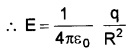# Plus Two Physics Notes Chapter 1 Electric Charges and Fields

Students can Download Chapter 1 Electric Charges and Fields Notes, Plus Two Physics Notes helps you to revise the complete Kerala State Syllabus and score more marks in your examinations.

## Kerala Plus Two Physics Notes Chapter 1 Electric Charges and Fields

Introduction
You may have seen a spark (or a crackle sound), when we take off our synthetic clothes. Have you ever tried to find any explanation for this phenomenon? Do you know the reason for lightning?

The above phenomenon can be explained on the basis of static electricity. Static means anything that does not change with time. Electrostatics deals with the properties of charges at rest.Electric Charge
It is found experimentally that the charges are of two types:

1. Positive charge
2. Negative change

The unit of charge is Coulomb (c).
Note: Positively charged body means deficiency of electrons in the body and a negatively charged body means excess of electrons.

Gold-Leaf electroscope: A simple apparatus to detect charge on a body is called a gold-leaf electroscope.

Apparatus: It consists of a vertical metal rod placed in a box. Two thin gold leaves are attached to its bottom end as shown in figure.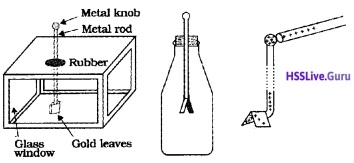Working: A charged object touches the metal knob at the top of the rod. Charge flows on to the leaves and they diverge. The degree of divergence is an indicator of the amount of charge.

Conductors And Insulators
Conductors: Conductors are those substances which allow passage of electricity through them.
Insulators: Insulators are those substances which do not allow passage of electricity through them.

1. Earthing (Or) Grounding:
When a charged body bring in contact with earth, all the excess charge pass to the earth through the connecting conductor. This process of sharing the charges with the earth is called grounding or earthing. Earthing provides protection to electrical circuits and appliances.Charging By Induction
A body can be charged in different ways.

1. Charging by friction
2. Charging by conduction
3. Charging by induction

1. Charging by friction:
When two bodies are rubbed each other, electronsin one body (in which electrons are held less tightly) transferred to second body (in which electrons are held more tightly).

Explanation: When a glass rod is rubbed with silk, some of the electrons from the glass are transferred to silk. Hence glass rod gets +ve charge and silk gets -ve charge.

2. Charging by conduction:
Charging a body with actual contact of another body is called charging by conduction.
Explanation:If a neutral conducting body (A) is brought in contact with positively charged conducting body (B), the neutral body gets positively charged.

3. Charging by induction:
The phenomenon by which a neutral body gets charged by the presence of neighboring charged body is called electrostatic induction.
Explanation:
Step I: Place two metal spheres on an insulating stand and bring in contact as shown in figure (a).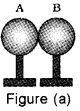Step II: Bring a positively charged rod near to these spheres. The free electrons in the spheres are attracted towards the rod. Hence, one side of the sphere becomes negative and the other side becomes positive as shown in the figure (b).Step III: Separate the spheres by a small distance by keeping the rod nearto sphere A. The two spheres are found to be oppositely charged as shown in figure (c).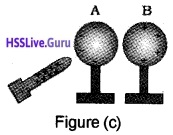Step IV: Remove the rod, the charge on spheres rearrange themselves as shown in figure (d).In this process, equal and opposite charges are developed on each sphere.

Basic Properties Of Electric Charge
1. Unlike charges attract and like charges repel.

2. Charge is conserved : Changes can neither be created nor be destroyed.
Explanation: When a glass rod is rubbed with silk, some of the electrons from the glass are transferred to silk. Hence glass rod gets +ve change and silk gets -ve changes.

3. Electric Charge is Quantized: Change on any body is the integral multiple of electronic charge. This is called quantization of charge.
i.e. q = ± ne, n = 1, 2, 3, ……….

4. Additivity of Charges: If a system contains n charges q1, q2, q3,……..qn, then the total change of the system is q1 + q2 + q3 +……+qn.

Coulomb’S Law
Statement: The force between two stationary electric charges is directly proportional to the product of the charges and inversely proportional to the square of the distance between them.
Explanation: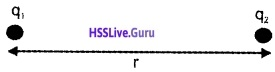Consider two point charges q1 and q1. which are separated by a distance ’r’. The force between the changes.
$$\mathrm{F}=\frac{1}{4 \pi \varepsilon_{0}} \frac{\mathrm{q}_{1} \mathrm{q}_{2}}{\mathrm{r}^{2}}$$
vector form: The force F12 (on the first charge by second} is given by (vector form)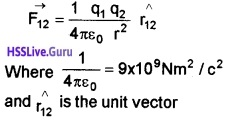Forces Between Multiple Charges
Super position principle: If the system contains a number of interacting changes, then the force on a given charge is equal to the vector sum of the forces exerted on it by all remaining charges.
Explanation: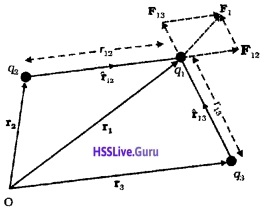Consider a system of three charges q1 q2 and q3 as shown in figure.
The force on q1 due to q2
$$\overrightarrow{F_{12}}=\frac{1}{4 \pi \varepsilon_{0} r_{12}^{2}} r_{12}^{\wedge}$$
Similarly the force q1 due to q3
$$\overrightarrow{F_{13}}=\frac{1}{4 \pi \varepsilon_{0}} \frac{q_{1} q_{2}}{r_{13}^{2}} r_{13}^{\wedge}$$
The total force F1 on q1 (due to q2 and q3) can be written as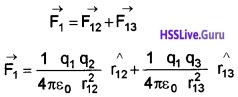System of ‘n’ charges: If system contains ‘n’ charges, total force acting on q1 due to all other charges.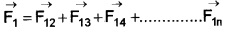Electric Field
The concept electric field is introduced to explain the interaction between two charges.
Electric field intensity: Strength or intensity of the electric field at any point is defined as the force acting on a unit positive charge placed at that point.
Mathematical expression of electric field intensity:Consider a charge q (test charge) at a distance ‘r’ from a source charge Q.
The force acting on q due to Q.
$$\mathrm{F}=\frac{1}{4 \pi \varepsilon_{0}} \frac{\mathrm{Qq}}{\mathrm{r}^{2}}$$
If q = 1, the force acting on this unit charge due to Q
$$\mathrm{F}=\frac{1}{4 \pi \varepsilon_{0}} \frac{\mathrm{Q}}{\mathrm{r}^{2}}$$
This force is called electric field intensity at a distance ‘r’ due to the charge Q.
ie; $$E=\frac{1}{4 \pi \varepsilon_{0}} \frac{Q}{r^{2}}$$

1. Electric field due to a system of charges:
Consider a system of charges q1, q2………..qn. Let P be point at distances r1p, r2p,………..rnp from charges. q1, q2,……..qn respectively. According to super position principle, total electric field at ‘p’ due to all other charges,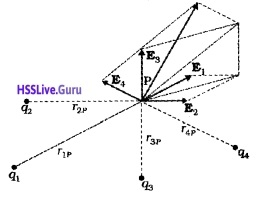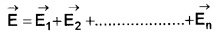2. Physical Significance Of Electric Field:
Question 1.
What are the importance of the concept of electric field?

• Electric field explains the electrical environment of a system of charges.
• Electric field help us to explain the interaction between two charges at rest or in motion.Electric Field Lines
Properties of Electric Lines of Force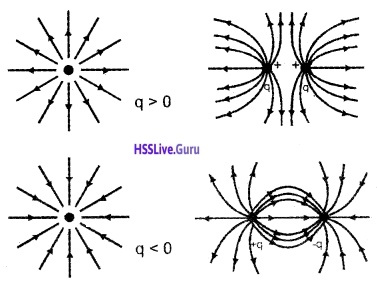(Field lines due to some simple charge configurations)

1. An electric line of force originates from positive charge and ends on negative charge.
2. The tangent drawn at a point on an electric line of force will give the direction of electric field at that point.
3. Two lines of force never intersect each other. (If they cut each other, at the point of intersection there will be two tangents. This indicates that there will be two directions of electric field at the same point which is impossible).
4. The number of electric lines of force passing normally through an area is directly proportional to the strength of the electric field.
5. The relative density of the field lines indicates the relative strength of electric field.
6. Electric field lines due to static charge never form closed loops.
7. In a uniform electric field, lines of force are parallel.

Electric Flux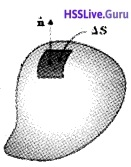Consider a closed surface. Let ∆$$\vec{S}$$ be a small area element on the surface. The electric field lines (E) passes through this area element at an angle θ. Electric flux ∆Φ through an area element ∆$$\vec{S}$$ is defined by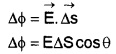The direction of area vector d$$\vec{S}$$ is perpendicular to the surface.Electric Dipole
Electric dipole: A pair of equal and opposite charges separated by small distance is called electric dipole. Dipole moment (p): Electric dipole moment (p) is defined as product of magnitude of charge and dipole length.
Dipole moment p = q × 2a
q – charge, 2a – dipole length
Dipole moment is a vector, directed from negative to positive charge.

1. Electric field at a point on the axial line of an electric dipole: Consider an electric dipole of moment P = 2aq. Let ‘S’ be a point at a distance ‘r’ from the centre of the dipole.Electric field at ‘S’ due to point charge at ‘A’Directed as shown in figure. Electric field at ‘S’ due to point charge at ‘B’Directed as shown in figure. Therefore resultant electric field at ‘S’
And its magnitude E = EB + -EAP = q × 2a
We can neglect a2 because a<<r.
∴ Electric field at S,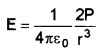This can be written in vector form as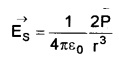The direction is along EB.
The field due to an electric dipole is directed from negative charge to positive charge along the axial line.

2. Electric field due to a dipole at a point on the perpendicular bisector of the dipole (at a point on the equatorial line).Consider a dipole of dipole moment P = 2aq. Let ‘S’ be a point on its equatorial line at a distance ‘r’ from its centre. The magnitudes of electric field at ‘S’ due to +q and -q are equal and acts as shown in figure. To find the resultant electric field resolve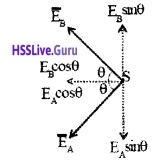Their normal components cancel each otherwhere as their horizontal components add up to give the resultant field at ‘S’.
E = EAcos θ + EBcos θ = 2 EB cos θ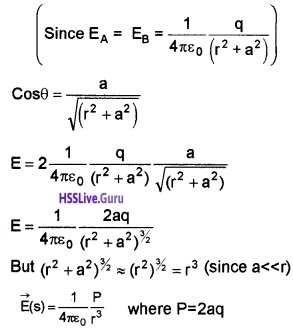The direction of the field due to the dipole at a point on the equatorial line is opposite to the direction of dipole moment.

3. Physical significance of dipole: The molecules of dielectrics may be classified into two classes:
(i) Polar molecules: In polar molecule, the centres of negative charges and positive charges do not coincide. Therefore they have a permanent
Example: H20, HCl, etc.

(ii) Nonpolar molecule: In nonpolar molecule, the centres of negative charges and positive charges coincide. Therefore they have no permanent electric dipole moment.
Example: C02, CH4, etc.
Note: In the presence of external electric field, a nonpolar molecule becomes a polar molecule.Dipole In A Uniform External FieldConsider an electric dipole of dipole moment P = 2aq kept in a uniform external electric field, inclined at an angle θ to the field direction.

Equal and opposite forces +qE and -qE act on the two charges. Hence the net force on the dipole is zero. But these two equal and opposite forces whose lines of action are different. Hence there will be a torque.
torque = any one force × perpendicular distance (between the line of action of two forces)
τ = qE × 2 a sin θ
Since P = 2aq
τ = P E sin θ
Vectorialy $$\vec{\tau}=\vec{P} \times \vec{E}$$
This torque tries to align the dipole along the direction of the external field.
Special Case:

• When θ = 0 ; τ =0
• When θ = 90; τ = PE, the maximum.

Note: In uniform electric field dipole has only rotational motion

Dipole in nonuniform electric field:
Question 2.
What happens to dipole if the applied electric field is nonuniform?
In nonuniform electric field, the net force and torque acting on the dipole will not be zero. Hence the dipole undergoes for both translational and rotational motion.

Continuous Charge Distribution
Charges on a body may be distributed in different ways according to the nature of body. Depending upon this distribution of charge, we deal with different types of charge densities,

1. Line charge density, λ
2. Surface charge density, σ or
3. Volume charge density, ρ

1. Linear charge density (λ): Charge per unit length is called linear charge density. If ∆Q is the charge contained in a line element ∆l,
Linear charge density λ = $$\frac{\Delta Q}{\Delta l}$$

2. Surface charge density (σ): Charge per unit area is called surface charge density. If ∆Q is the charge contained in a area element ∆s, surface charge density can be written as
σ = $$\frac{\Delta Q}{\Delta S}$$

3. Volume charge density (ρ): Charge per unit volume is called volume change density. If ∆Q is the charge contained in a volume ∆v, volume charge density.
ρ = $$\frac{\Delta Q}{\Delta v}$$Gauss’s Law
Gauss’s theorem states that the total electric flux over a closed surface is 1/ε0 times the total charge enclosed by the surface.
Gauss’s theorem may be expressed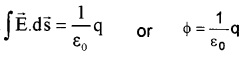Proof: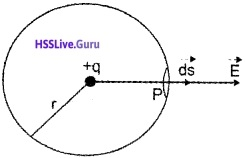Consider a charge +q .which is kept inside a sphere of radius ‘r’.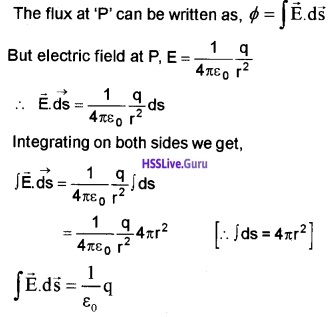Important points regarding Gauss’s law:

• Gauss’s law is true for any closed surface.
• Total charge enclosed by the surface must be added (algebraically). The charge may be located anywhere inside the surface.
• The surface that we choose for the application of Gauss’s law is called the Gaussian surface.
• Gauss’s law is used to find electric field due to system of charges having some symmetry.
Gauss’s law is based on the inverse square of distance. Any violation of Gauss’s law will indicate departure from the inverse square law.

Applications Of Gauss’s Law
Gauss’s law can be used to find electric field due to system of some symmetric charge configurations. Some examples are given below.

1. Field Due To An Infinitely Long Straight Uniformly Charged Wire:Consider a thin infinitely long straight rod conductor having change density λ. (λ = $$\frac{q}{l}$$)
To find the electric field at P, we imagine a Gaussian surface passing through P.
Then according to Gauss’s law we can write,Integrating over the Gaussian surface, we get (we need not integrate the upper and lower surface because, electric lines do not pass through these surfaces.)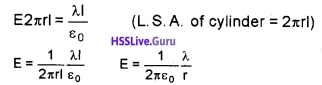2. Field Due To A Uniformly Charged Infinite Plane Sheet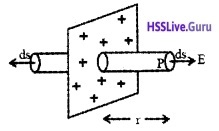Consider an infinite thin plane sheet of change of density σ. To find electric field at a point P (at a distance ‘r’ from sheet), imagine a Gaussian surface in the form of cylinder having area of cross section ‘ds’.
According to Gauss’s law we can write,(Since q = σds)
But electric field passes only through end surfaces ,so we get ∫ds = 2ds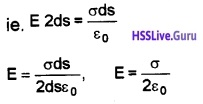E is directed away from the charged sheet, if a is positive and directed towards the sheet if a is negative.

3. Field Due To A Uniformly Charged Thin Spherical Shell: Consider a uniformly changed hollow spherical conductor of radius R. Let ‘q’ be the total charge on the surface.To find the electric field at P (at a distance r from the centre), we imagine a Gaussian spherical surface having radius ‘r’.
Then, according to Gauss’s theorem we can write,The electric field is constant, at a distance ‘r’. So we can write,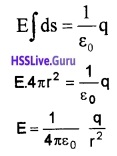Case I: Electric field inside the shell is zero.
Case II: At the surface of shell r = R